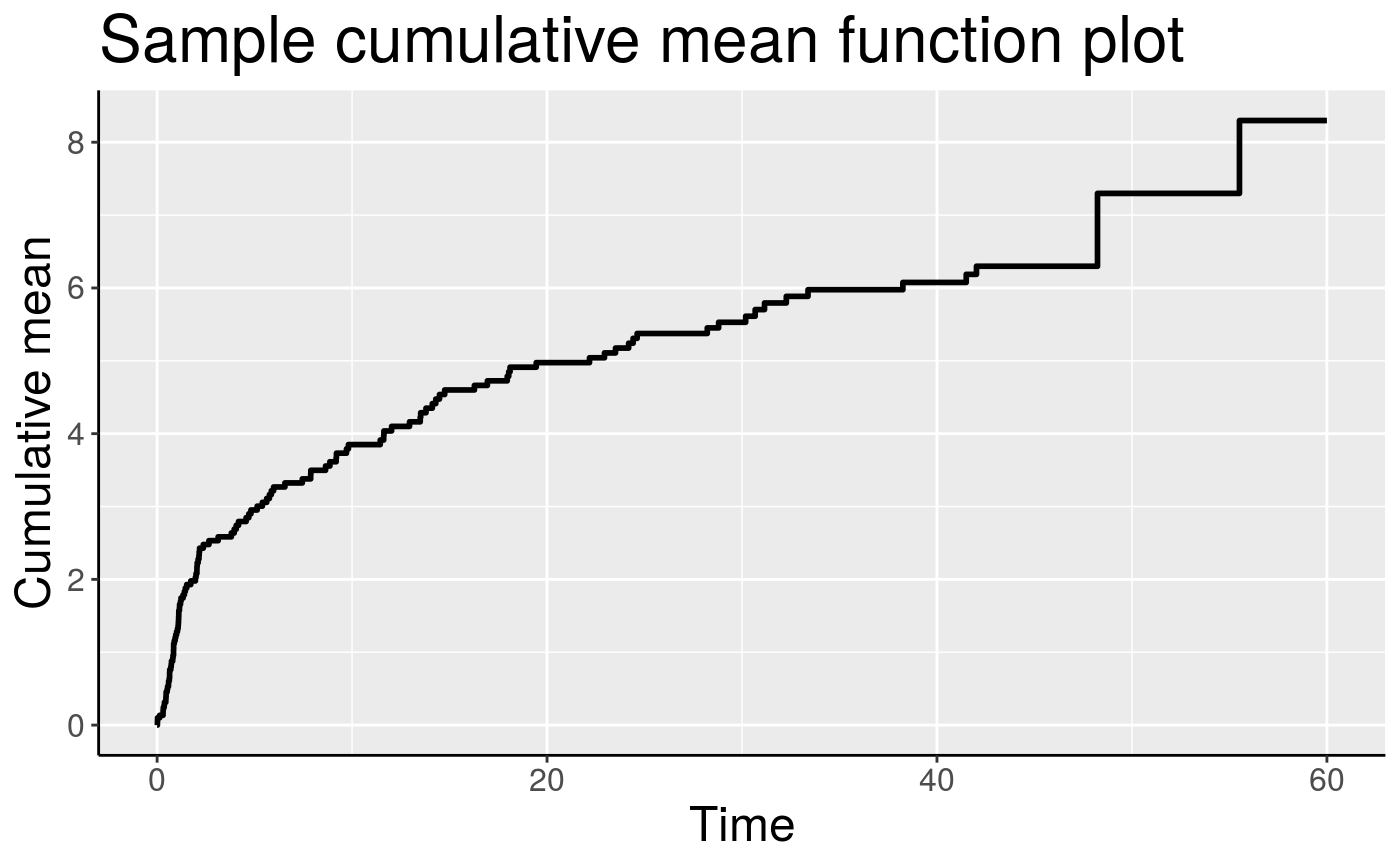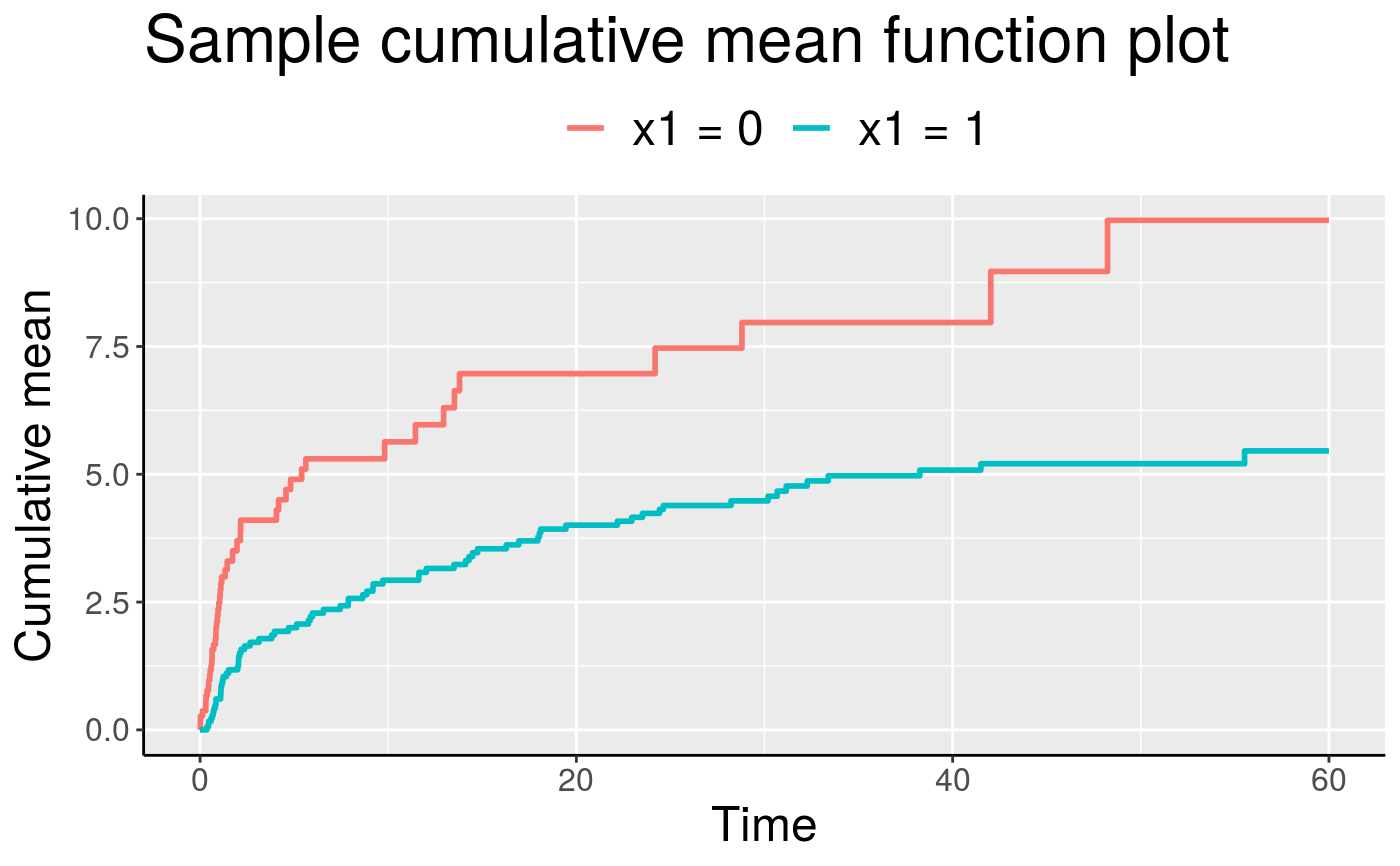Plot the mean cumulative function (MCF) for an Recur object. The usage of the function is similar to that of plot.Recur() but with more flexible options.

plotMCF(
formula,
data,
adjrisk = TRUE,
onePanel = FALSE,
smooth = FALSE,
control = list(),
...
)

## Arguments

formula a formula object, with the response on the left of a "~" operator, and the predictors on the right. The response must be a recurrent event survival object returned by the Recur() function. an optional data frame in which to interpret the variables occurring in the "formula". an optional logical value that is passed to the plotMCF() function as the adjrisk argument. See plotMCF. This argument indicates whether risk set size will be adjusted. If mcf.adjrisk = TRUE, subjects leave the risk set after terminal times as in the Nelson-Aalen estimator. If mcf.adjrisk = FALSE, subjects remain in the risk set after terminal time. an optional logical value indicating whether the mean cumulative functions will be plotted in the same panel. This is only useful when there are multiple recurrent event types or in the presence of (discrete) covariates. an optional logical value indicating whether to add a smooth curve obtained from a monotone increasing P-splines implemented in package scam. This feature only works for data with one recurrent event type. a list of control parameters. graphical parameters to be passed to methods. These include xlab, ylab, main, and more. See Details.

## Value

A ggplot object.

## Details

When adjrisk = TRUE, the plotMCF() is equivalent to the Nelson-Aalen estimator for the intensity function of the recurrent event process. When adjrisk = FALSE, the plotMCF() does not adjust for the risk set and assumes all subjects remain at risk after the last observed recurrent event. This is known as the survivor rate function. The argument control consists of options with argument defaults to a list with the following values:

xlab

customizable x-label, default value is "Time".

ylab

customizable y-label, default value is "Cumulative mean".

main

customizable title, default value is "Sample cumulative mean function plot".

The xlab, ylab and main parameters can also be passed down without specifying a control list.

## See also

Recur, plot.Recur

## Examples

data(simDat)
plotMCF(Recur(t.stop, id, event, status) ~ 1, data = simDat)plotMCF(Recur(t.stop, id, event, status) ~ x1, data = simDat, onePanel = TRUE)